Calculus Volume 2

# Chapter 1

### Checkpoint

1.1

$∑ i = 3 6 2 i = 2 3 + 2 4 + 2 5 + 2 6 = 120 ∑ i = 3 6 2 i = 2 3 + 2 4 + 2 5 + 2 6 = 120$

1.2

15,550

1.3

440

1.4

The left-endpoint approximation is 0.7595. The right-endpoint approximation is 0.6345. See the below [link].1.5
1. $Upper sum=8.0313.Upper sum=8.0313.$

2.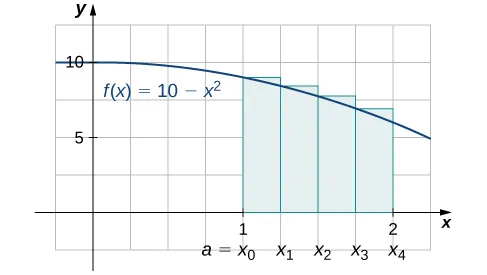1.6

$A ≈ 1.125 A ≈ 1.125$

1.7

6

1.8

18 square units

1.9

6

1.10

18

1.11

$6 ∫ 1 3 x 3 d x − 4 ∫ 1 3 x 2 d x + 2 ∫ 1 3 x d x − ∫ 1 3 3 d x 6 ∫ 1 3 x 3 d x − 4 ∫ 1 3 x 2 d x + 2 ∫ 1 3 x d x − ∫ 1 3 3 d x$

1.12

−7

1.13

3

1.14

$Average value = 1.5 ; c = 3 Average value = 1.5 ; c = 3$

1.15

$c = 3 c = 3$

1.16

$g ′ ( r ) = r 2 + 4 g ′ ( r ) = r 2 + 4$

1.17

$F ′ ( x ) = 3 x 2 cos x 3 F ′ ( x ) = 3 x 2 cos x 3$

1.18

$F ′ ( x ) = 2 x cos x 2 − cos x F ′ ( x ) = 2 x cos x 2 − cos x$

1.19

$7 24 7 24$

1.20

Kathy still wins, but by a much larger margin: James skates 24 ft in 3 sec, but Kathy skates 29.3634 ft in 3 sec.

1.21

$− 10 3 − 10 3$

1.22

Net displacement: $e2−92≈−0.8055m;e2−92≈−0.8055m;$ total distance traveled: $4ln4−7.5+e22≈1.7404ln4−7.5+e22≈1.740$ m

1.23

17.5 mi

1.24

$64 5 64 5$

1.25

$∫ 3 x 2 ( x 3 − 3 ) 2 d x = 1 3 ( x 3 − 3 ) 3 + C ∫ 3 x 2 ( x 3 − 3 ) 2 d x = 1 3 ( x 3 − 3 ) 3 + C$

1.26

$( x 3 + 5 ) 10 30 + C ( x 3 + 5 ) 10 30 + C$

1.27

$− 1 sin t + C − 1 sin t + C$

1.28

$− cos 4 t 4 + C − cos 4 t 4 + C$

1.29

$91 3 91 3$

1.30

$2 3 π ≈ 0.2122 2 3 π ≈ 0.2122$

1.31

$∫ x 2 e −2 x 3 d x = − 1 6 e −2 x 3 + C ∫ x 2 e −2 x 3 d x = − 1 6 e −2 x 3 + C$

1.32

$∫ e x ( 3 e x − 2 ) 2 d x = 1 9 ( 3 e x − 2 ) 3 ∫ e x ( 3 e x − 2 ) 2 d x = 1 9 ( 3 e x − 2 ) 3$

1.33

$∫ 2 x 3 e x 4 d x = 1 2 e x 4 ∫ 2 x 3 e x 4 d x = 1 2 e x 4$

1.34

$1 2 ∫ 0 4 e u d u = 1 2 ( e 4 − 1 ) 1 2 ∫ 0 4 e u d u = 1 2 ( e 4 − 1 )$

1.35

$Q(t)=2tln2+8.557.Q(t)=2tln2+8.557.$ There are 20,099 bacteria in the dish after 3 hours.

1.36

There are 116 flies.

1.37

$∫ 1 2 1 x 3 e 4 x −2 d x = 1 8 [ e 4 − e ] ∫ 1 2 1 x 3 e 4 x −2 d x = 1 8 [ e 4 − e ]$

1.38

$ln | x + 2 | + C ln | x + 2 | + C$

1.39

$x ln 3 ( ln x − 1 ) + C x ln 3 ( ln x − 1 ) + C$

1.40

$1 4 sin −1 ( 4 x ) + C 1 4 sin −1 ( 4 x ) + C$

1.41

$sin −1 ( x 3 ) + C sin −1 ( x 3 ) + C$

1.42

$1 10 tan −1 ( 2 x 5 ) + C 1 10 tan −1 ( 2 x 5 ) + C$

1.43

$1 4 tan −1 ( x 4 ) + C 1 4 tan −1 ( x 4 ) + C$

1.44

$π 8 π 8$

### Section 1.1 Exercises

1.

a. They are equal; both represent the sum of the first 10 whole numbers. b. They are equal; both represent the sum of the first 10 whole numbers. c. They are equal by substituting $j=i−1.j=i−1.$ d. They are equal; the first sum factors the terms of the second.

3.

$385 − 30 = 355 385 − 30 = 355$

5.

$15 − ( −12 ) = 27 15 − ( −12 ) = 27$

7.

$5 ( 15 ) + 4 ( −12 ) = 27 5 ( 15 ) + 4 ( −12 ) = 27$

9.

$∑ j = 1 50 j 2 − 2 ∑ j = 1 50 j = ( 50 ) ( 51 ) ( 101 ) 6 − 2 ( 50 ) ( 51 ) 2 = 40 , ​ 375 ∑ j = 1 50 j 2 − 2 ∑ j = 1 50 j = ( 50 ) ( 51 ) ( 101 ) 6 − 2 ( 50 ) ( 51 ) 2 = 40 , ​ 375$

11.

$4 ∑ k = 1 25 k 2 − 100 ∑ k = 1 25 k = 4 ( 25 ) ( 26 ) ( 51 ) 6 − 50 ( 25 ) ( 26 ) = −10 , ​ 400 4 ∑ k = 1 25 k 2 − 100 ∑ k = 1 25 k = 4 ( 25 ) ( 26 ) ( 51 ) 6 − 50 ( 25 ) ( 26 ) = −10 , ​ 400$

13.

$R 4 = -0.25 R 4 = -0.25$

15.

$R 6 = 0.372 R 6 = 0.372$

17.

$L 4 = 2.20 L 4 = 2.20$

19.

$L 8 = 0.6875 L 8 = 0.6875$

21.

$L6=9.000=R6.L6=9.000=R6.$ The graph of f is a triangle with area 9.

23.

$L6=13.12899=R6.L6=13.12899=R6.$ They are equal.

25.

$L 10 = 4 10 ∑ i = 1 10 4 − ( −2 + 4 ( i − 1 ) 10 ) 2 L 10 = 4 10 ∑ i = 1 10 4 − ( −2 + 4 ( i − 1 ) 10 ) 2$

27.

$R 100 = e − 1 100 ∑ i = 1 100 ln ( 1 + ( e − 1 ) i 100 ) R 100 = e − 1 100 ∑ i = 1 100 ln ( 1 + ( e − 1 ) i 100 )$

29.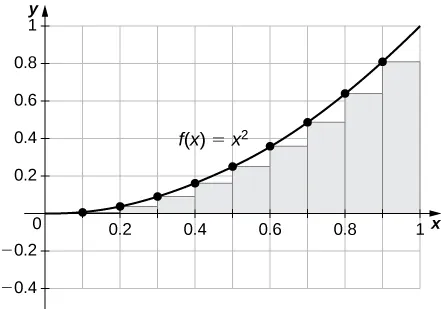$R100=0.33835,L100=0.32835.R100=0.33835,L100=0.32835.$ The plot shows that the left Riemann sum is an underestimate because the function is increasing. Similarly, the right Riemann sum is an overestimate. The area lies between the left and right Riemann sums. Ten rectangles are shown for visual clarity. This behavior persists for more rectangles.

31.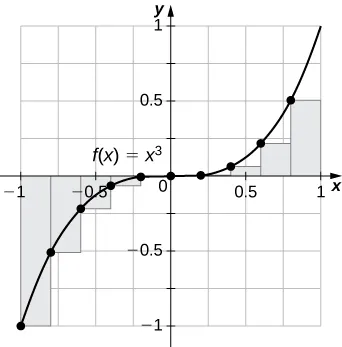$L100=−0.02,R100=0.02.L100=−0.02,R100=0.02.$ The left endpoint sum is an underestimate because the function is increasing. Similarly, a right endpoint approximation is an overestimate. The area lies between the left and right endpoint estimates.

33.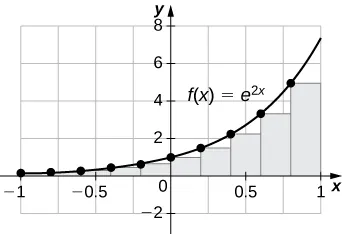$L100=3.980,R100=4.020,R100>L100L100=3.980,R100=4.020,R100>L100$ The plot shows that the left Riemann sum is an underestimate because the function is increasing. Ten rectangles are shown for visual clarity. This behavior persists for more rectangles.

35.

The sum represents the cumulative rainfall in January 2009.

37.

The total mileage is $7×∑i=125(1+(i−1)10)=7×25+710×12×25=385mi.7×∑i=125(1+(i−1)10)=7×25+710×12×25=385mi.$

39.

Add the numbers to get 8.1-in. net increase.

41.

309,389,957

43.

$L 8 = 3 + 2 + 1 + 2 + 3 + 4 + 5 + 4 = 24 L 8 = 3 + 2 + 1 + 2 + 3 + 4 + 5 + 4 = 24$

45.

$L 8 = 3 + 5 + 7 + 6 + 8 + 6 + 5 + 4 = 44 L 8 = 3 + 5 + 7 + 6 + 8 + 6 + 5 + 4 = 44$

47.

$L 10 ≈ 1.7604 , L 30 ≈ 1.7625 , L 50 ≈ 1.76265 L 10 ≈ 1.7604 , L 30 ≈ 1.7625 , L 50 ≈ 1.76265$

49.

$R1=−1,L1=1,R10=−0.1,L10=0.1,L100=0.01,R1=−1,L1=1,R10=−0.1,L10=0.1,L100=0.01,$ and $R100=−0.1.R100=−0.1.$ By symmetry of the graph, the exact area is zero.

51.

$R 1 = 0 , L 1 = 0 , R 10 = 2.4499 , L 10 = 2.4499 , R 100 = 2.1365 , L 100 = 2.1365 R 1 = 0 , L 1 = 0 , R 10 = 2.4499 , L 10 = 2.4499 , R 100 = 2.1365 , L 100 = 2.1365$

53.

If $[c,d][c,d]$ is a subinterval of $[a,b][a,b]$ under one of the left-endpoint sum rectangles, then the area of the rectangle contributing to the left-endpoint estimate is $f(c)(d−c).f(c)(d−c).$ But, $f(c)≤f(x)f(c)≤f(x)$ for $c≤x≤d,c≤x≤d,$ so the area under the graph of f between c and d is $f(c)(d−c)f(c)(d−c)$ plus the area below the graph of f but above the horizontal line segment at height $f(c),f(c),$ which is positive. As this is true for each left-endpoint sum interval, it follows that the left Riemann sum is less than or equal to the area below the graph of f on $[a,b].[a,b].$

55.

$LN=b−aN∑i=1Nf(a+(b−a)i−1N)=b−aN∑i=0N−1f(a+(b−a)iN)LN=b−aN∑i=1Nf(a+(b−a)i−1N)=b−aN∑i=0N−1f(a+(b−a)iN)$ and $RN=b−aN∑i=1Nf(a+(b−a)iN).RN=b−aN∑i=1Nf(a+(b−a)iN).$ The left sum has a term corresponding to $i=0i=0$ and the right sum has a term corresponding to $i=N.i=N.$ In $RN−LN,RN−LN,$ any term corresponding to $i=1,2,…,N−1i=1,2,…,N−1$ occurs once with a plus sign and once with a minus sign, so each such term cancels and one is left with $RN−LN=b−aN(f(a+(b−a))NN)−(f(a)+(b−a)0N)=b−aN(f(b)−f(a)).RN−LN=b−aN(f(a+(b−a))NN)−(f(a)+(b−a)0N)=b−aN(f(b)−f(a)).$

57.

Graph 1: a. $L(A)=0,B(A)=20;L(A)=0,B(A)=20;$ b. $U(A)=20.U(A)=20.$ Graph 2: a. $L(A)=9;L(A)=9;$ b. $B(A)=11,U(A)=20.B(A)=11,U(A)=20.$ Graph 3: a. $L(A)=11.0;L(A)=11.0;$ b. $B(A)=4.5,U(A)=15.5.B(A)=4.5,U(A)=15.5.$

59.

Let A be the area of the unit circle. The circle encloses n congruent triangles each of area $sin(2πn)2,sin(2πn)2,$ so $n2sin(2πn)≤A.n2sin(2πn)≤A.$ Similarly, the circle is contained inside n congruent triangles each of area $BH2=12cosπn+sinπntanπn2sin2πn,BH2=12cosπn+sinπntanπn2sin2πn,$ so $A≤n2sin(2πn)(cos(πn)+sin(πn)tan(πn))2.A≤n2sin(2πn)(cos(πn)+sin(πn)tan(πn))2.$ As $n→∞,n2sin(2πn)=sin(2πn)(2n)Α→π,n→∞,n2sin(2πn)=sin(2πn)(2n)Α→π,$ so we conclude $π≤A.π≤A.$ Also, as $n→∞,cos(πn)+sin(πn)tan(πn)→1,n→∞,cos(πn)+sin(πn)tan(πn)→1,$ so we also have $A≤π.A≤π.$ By the squeeze theorem for limits, we conclude that $A=π.A=π.$

### Section 1.2 Exercises

61.

$∫ 0 2 ( 5 x 2 − 3 x 3 ) d x ∫ 0 2 ( 5 x 2 − 3 x 3 ) d x$

63.

$∫ 0 1 cos 2 ( 2 π x ) d x ∫ 0 1 cos 2 ( 2 π x ) d x$

65.

$∫ 0 1 x d x ∫ 0 1 x d x$

67.

$∫ 3 6 x d x ∫ 3 6 x d x$

69.

$∫ 1 2 x log ( x 2 ) d x ∫ 1 2 x log ( x 2 ) d x$

71.

$1 + 2 · 2 + 3 · 3 = 14 1 + 2 · 2 + 3 · 3 = 14$

73.

$1 − 4 + 9 = 6 1 − 4 + 9 = 6$

75.

$1 − 2 π + 9 = 10 − 2 π 1 − 2 π + 9 = 10 − 2 π$

77.

The integral is the area of the triangle, $1212$

79.

The integral is the area of the triangle, 9.

81.

The integral is the area $12πr2=2π.12πr2=2π.$

83.

The integral is the area of the “big” triangle less the “missing” triangle, $9−12.9−12.$

85.

$L = 2 + 0 + 10 + 5 + 4 = 21 , R = 0 + 10 + 10 + 2 + 0 = 22 , L + R 2 = 21.5 L = 2 + 0 + 10 + 5 + 4 = 21 , R = 0 + 10 + 10 + 2 + 0 = 22 , L + R 2 = 21.5$

87.

$L = 0 + 4 + 0 + 4 + 2 = 10 , R = 4 + 0 + 2 + 4 + 0 = 10 , L + R 2 = 10 L = 0 + 4 + 0 + 4 + 2 = 10 , R = 4 + 0 + 2 + 4 + 0 = 10 , L + R 2 = 10$

89.

$∫ 2 4 f ( x ) d x + ∫ 2 4 g ( x ) d x = 8 − 3 = 5 ∫ 2 4 f ( x ) d x + ∫ 2 4 g ( x ) d x = 8 − 3 = 5$

91.

$∫ 2 4 f ( x ) d x − ∫ 2 4 g ( x ) d x = 8 + 3 = 11 ∫ 2 4 f ( x ) d x − ∫ 2 4 g ( x ) d x = 8 + 3 = 11$

93.

$4 ∫ 2 4 f ( x ) d x − 3 ∫ 2 4 g ( x ) d x = 32 + 9 = 41 4 ∫ 2 4 f ( x ) d x − 3 ∫ 2 4 g ( x ) d x = 32 + 9 = 41$

95.

The integrand is odd; the integral is zero.

97.

The integrand is antisymmetric with respect to $x=3.x=3.$ The integral is zero.

99.

$1 − 1 2 + 1 3 − 1 4 = 7 12 1 − 1 2 + 1 3 − 1 4 = 7 12$

101.

$∫ 0 1 ( 1 − 6 x + 12 x 2 − 8 x 3 ) d x = ( x - 3 x 2 + 4 x 3 - 2 x 4 ) = ( 1 - 3 + 4 - 2 ) ( 0 - 0 + 0 - 0 ) = 0 ∫ 0 1 ( 1 − 6 x + 12 x 2 − 8 x 3 ) d x = ( x - 3 x 2 + 4 x 3 - 2 x 4 ) = ( 1 - 3 + 4 - 2 ) ( 0 - 0 + 0 - 0 ) = 0$

103.

$7 − 5 4 = 23 4 7 − 5 4 = 23 4$

105.

The integrand is negative over $[−2,3].[−2,3].$

107.

$x≤x2x≤x2$ over $[1,2],[1,2],$ so $1+x≤1+x21+x≤1+x2$ over $[1,2].[1,2].$

109.

$cos(t)≥22.cos(t)≥22.$ Multiply by the length of the interval to get the inequality.

111.

$f ave = 0 ; c = 0 f ave = 0 ; c = 0$

113.

$3232$ when $c=±32c=±32$

115.

$f ave = 0 ; c = π 2 , 3 π 2 f ave = 0 ; c = π 2 , 3 π 2$

117.

$L100=1.294,R100=1.301;L100=1.294,R100=1.301;$ the exact average is between these values.

119.

$L 100 × ( 1 2 ) = 0.5178 , R 100 × ( 1 2 ) = 0.5294 L 100 × ( 1 2 ) = 0.5178 , R 100 × ( 1 2 ) = 0.5294$

121.

$L1=0,L10×(12)=8.743493,L100×(12)=12.861728.L1=0,L10×(12)=8.743493,L100×(12)=12.861728.$ The exact answer $≈26.799,≈26.799,$ so L100 is not accurate.

123.

$L1×(1π)=1.352,L10×(1π)=−0.1837,L100×(1π)=−0.2956.L1×(1π)=1.352,L10×(1π)=−0.1837,L100×(1π)=−0.2956.$ The exact answer $≈−0.303,≈−0.303,$ so L100 is not accurate to first decimal.

125.

Use $tan2θ+1=sec2θ.tan2θ+1=sec2θ.$ Then, $B−A=∫−π/4π/41dx=π2.B−A=∫−π/4π/41dx=π2.$

127.

$∫02πcos2tdt=π,∫02πcos2tdt=π,$ so divide by the length 2π of the interval. $cos2tcos2t$ has period π, so yes, it is true.

129.

The integral is maximized when one uses the largest interval on which p is nonnegative. Thus, $A=−b−b2−4ac2aA=−b−b2−4ac2a$ and $B=−b+b2−4ac2a.B=−b+b2−4ac2a.$

131.

If $f(t0)>g(t0)f(t0)>g(t0)$ for some $t0∈[a,b],t0∈[a,b],$ then since $f−gf−g$ is continuous, there is an interval containing t0 such that $f(t)>g(t)f(t)>g(t)$ over the interval $[c,d],[c,d],$ and then $∫ddf(t)dt>∫cdg(t)dt∫ddf(t)dt>∫cdg(t)dt$ over this interval.

133.

The integral of f over an interval is the same as the integral of the average of f over that interval. Thus, $∫abf(t)dt=∫a0a1f(t)dt+∫a1a2f(t)dt+⋯+∫aN+1aNf(t)dt=∫a0a11dt+∫a1a21dt+⋯+∫aN+1aN1dt=(a1−a0)+(a2−a1)+⋯+(aN−aN−1)=aN−a0=b−a.∫abf(t)dt=∫a0a1f(t)dt+∫a1a2f(t)dt+⋯+∫aN+1aNf(t)dt=∫a0a11dt+∫a1a21dt+⋯+∫aN+1aN1dt=(a1−a0)+(a2−a1)+⋯+(aN−aN−1)=aN−a0=b−a.$ Dividing through by $b−ab−a$ gives the desired identity.

135.

$∫ 0 N f ( t ) d t = ∑ i = 1 N ∫ i − 1 i f ( t ) d t = ∑ i = 1 N i 2 = N ( N + 1 ) ( 2 N + 1 ) 6 ∫ 0 N f ( t ) d t = ∑ i = 1 N ∫ i − 1 i f ( t ) d t = ∑ i = 1 N i 2 = N ( N + 1 ) ( 2 N + 1 ) 6$

137.

$L10=1.815,R10=1.515,L10+R102=1.665,L10=1.815,R10=1.515,L10+R102=1.665,$ so the estimate is accurate to two decimal places.

139.

The average is $1/2,1/2,$ which is equal to the integral in this case.

141.

a. The graph is antisymmetric with respect to $t=12t=12$ over $[0,1],[0,1],$ so the average value is zero. b. For any value of a, the graph between $[a,a+1][a,a+1]$ is a shift of the graph over $[0,1],[0,1],$ so the net areas above and below the axis do not change and the average remains zero.

143.

Yes, the integral over any interval of length 1 is the same.

### Section 1.3 Exercises

145.

Yes. It is implied by the Mean Value Theorem for Integrals.

147.

$F′(2)=−1;F′(2)=−1;$ average value of $F′F′$ over $[1,2][1,2]$ is $−1/2.−1/2.$

149.

$e cos x e cos x$

151.

$1 16 − x 2 1 16 − x 2$

153.

$x d d x x = 1 2 x d d x x = 1 2$

155.

$− 1 − cos 2 x d d x cos x = | sin x | sin x − 1 − cos 2 x d d x cos x = | sin x | sin x$

157.

$2 x | x | 1 + x 2 2 x | x | 1 + x 2$

159.

$ln ( e 2 x ) d d x e x = 2 x e x ln ( e 2 x ) d d x e x = 2 x e x$

161.

a. f is positive over $(1,2)(1,2)$ and $(5,6),(5,6),$ negative over $(0,1)(0,1)$ and $(3,4),(3,4),$ and zero over $(2,3)(2,3)$ and $(4,5).(4,5).$ b. The maximum value is 2 and the minimum is −3. c. The average value is 0.

163.

a. is positive over $(0,1)(0,1)$ and $(3,6),(3,6),$ and negative over $(1,3).(1,3).$ b. It is increasing over $(0,1)(0,1)$ and $(3,5),(3,5),$ and it is constant over $(1,3)(1,3)$ and $(5,6).(5,6).$ c. Its average value is $13.13.$

165.

$T 10 = 49.08 , ∫ −4 3 ( x 3 + 6 x 2 + x − 5 ) d x = 48 T 10 = 49.08 , ∫ −4 3 ( x 3 + 6 x 2 + x − 5 ) d x = 48$

167.

$T 10 = 260.836 , ∫ 1 9 ( x + x 2 ) d x = 260 T 10 = 260.836 , ∫ 1 9 ( x + x 2 ) d x = 260$

169.

$T 10 = 3.058 , ∫ 1 4 4 x 2 d x = 3 T 10 = 3.058 , ∫ 1 4 4 x 2 d x = 3$

171.

$F ( x ) = x 3 3 + 3 x 2 2 − 5 x , F ( 3 ) − F ( −2 ) = − 35 6 F ( x ) = x 3 3 + 3 x 2 2 − 5 x , F ( 3 ) − F ( −2 ) = − 35 6$

173.

$F ( x ) = − t 5 5 + 13 t 3 3 − 36 t , F ( 3 ) − F ( 2 ) = 62 15 F ( x ) = − t 5 5 + 13 t 3 3 − 36 t , F ( 3 ) − F ( 2 ) = 62 15$

175.

$F ( x ) = x 100 100 , F ( 1 ) − F ( 0 ) = 1 100 F ( x ) = x 100 100 , F ( 1 ) − F ( 0 ) = 1 100$

177.

$F ( x ) = x 3 3 + 1 x , F ( 4 ) − F ( 1 4 ) = 1125 64 F ( x ) = x 3 3 + 1 x , F ( 4 ) − F ( 1 4 ) = 1125 64$

179.

$F ( x ) = x , F ( 4 ) − F ( 1 ) = 1 F ( x ) = x , F ( 4 ) − F ( 1 ) = 1$

181.

$F ( x ) = 4 3 t 3 / 4 , F ( 16 ) − F ( 1 ) = 28 3 F ( x ) = 4 3 t 3 / 4 , F ( 16 ) − F ( 1 ) = 28 3$

183.

$F ( x ) = − cos x , F ( π 2 ) − F ( 0 ) = 1 F ( x ) = − cos x , F ( π 2 ) − F ( 0 ) = 1$

185.

$F ( x ) = sec x , F ( π 4 ) − F ( 0 ) = 2 − 1 F ( x ) = sec x , F ( π 4 ) − F ( 0 ) = 2 − 1$

187.

$F ( x ) = − cot ( x ) , F ( π 2 ) − F ( π 4 ) = 1 F ( x ) = − cot ( x ) , F ( π 2 ) − F ( π 4 ) = 1$

189.

$F ( x ) = − 1 x + 1 2 x 2 , F ( −1 ) − F ( −2 ) = 7 8 F ( x ) = − 1 x + 1 2 x 2 , F ( −1 ) − F ( −2 ) = 7 8$

191.

$F ( x ) = e x − e F ( x ) = e x − e$

193.

$F ( x ) = 0 F ( x ) = 0$

195.

$∫ −2 −1 ( t 2 − 2 t − 3 ) d t − ∫ −1 3 ( t 2 − 2 t − 3 ) d t + ∫ 3 4 ( t 2 − 2 t − 3 ) d t = 46 3 ∫ −2 −1 ( t 2 − 2 t − 3 ) d t − ∫ −1 3 ( t 2 − 2 t − 3 ) d t + ∫ 3 4 ( t 2 − 2 t − 3 ) d t = 46 3$

197.

$− ∫ − π / 2 0 sin t d t + ∫ 0 π / 2 sin t d t = 2 − ∫ − π / 2 0 sin t d t + ∫ 0 π / 2 sin t d t = 2$

199.

a. The average is $11.21×10911.21×109$ since $cos(πt6)cos(πt6)$ has period 12 and integral 0 over any period. Consumption is equal to the average when $cos(πt6)=0,cos(πt6)=0,$ when $t=3,t=3,$ and when $t=9.t=9.$ b. Total consumption is the average rate times duration: $11.21×12×109=1.35×101111.21×12×109=1.35×1011$ c. $109(11.21−16∫39cos(πt6)dt)=109(11.21+2π)=11.84x109109(11.21−16∫39cos(πt6)dt)=109(11.21+2π)=11.84x109$

201.

If f is not constant, then its average is strictly smaller than the maximum and larger than the minimum, which are attained over $[a,b][a,b]$ by the extreme value theorem.

203.

a. $d2θ=(acosθ+c)2+b2sin2θ=a2+c2cos2θ+2accosθ=(a+ccosθ)2;d2θ=(acosθ+c)2+b2sin2θ=a2+c2cos2θ+2accosθ=(a+ccosθ)2;$ b. $d–=12π∫02π(a+2ccosθ)dθ=ad–=12π∫02π(a+2ccosθ)dθ=a$

205.

Mean gravitational force = $GmM2π ∫02π 1 (a+2a2−b2cosθ)2 dθ. GmM2π ∫02π 1 (a+2a2−b2cosθ)2 dθ.$

### Section 1.4 Exercises

207.

$∫ ( x − 1 x ) d x = ∫ x 1 / 2 d x − ∫ x −1 / 2 d x = 2 3 x 3 / 2 + C 1 − 2 x 1 / 2 + C 2 = 2 3 x 3 / 2 − 2 x 1 / 2 + C ∫ ( x − 1 x ) d x = ∫ x 1 / 2 d x − ∫ x −1 / 2 d x = 2 3 x 3 / 2 + C 1 − 2 x 1 / 2 + C 2 = 2 3 x 3 / 2 − 2 x 1 / 2 + C$

209.

$∫ d x 2 x = 1 2 ln | x | + C ∫ d x 2 x = 1 2 ln | x | + C$

211.

$∫ 0 π sin x d x − ∫ 0 π cos x d x = − cos x | 0 π − ( sin x ) | 0 π = ( − ( −1 ) + 1 ) − ( 0 − 0 ) = 2 ∫ 0 π sin x d x − ∫ 0 π cos x d x = − cos x | 0 π − ( sin x ) | 0 π = ( − ( −1 ) + 1 ) − ( 0 − 0 ) = 2$

213.

$P(s)=4s,P(s)=4s,$ so $dPds=4dPds=4$ and $∫244ds=8.∫244ds=8.$

215.

$∫ 1 2 N d s = N ∫ 1 2 N d s = N$

217.

With p as in the previous exercise, each of the 12 pentagons increases in area from 2p to 4p units so the net increase in the area of the dodecahedron is 36p units.

219.

$18 s 2 = 6 ∫ s 2 s 2 x d x 18 s 2 = 6 ∫ s 2 s 2 x d x$

221.

$12 π R 2 = 8 π ∫ R 2 R r d r 12 π R 2 = 8 π ∫ R 2 R r d r$

223.

$d(t)=∫0tv(s)ds=4t−t2.d(t)=∫0tv(s)ds=4t−t2.$ The total distance is $d(2)=4m.d(2)=4m.$

225.

$d(t)=∫0tv(s)ds.d(t)=∫0tv(s)ds.$ For $t<3,d(t)=∫0t(6−2t)dt=6t−t2.t<3,d(t)=∫0t(6−2t)dt=6t−t2.$ For $t>3,d(t)=d(3)+∫3t(2t−6)dt=9+(t2−6t)|36.t>3,d(t)=d(3)+∫3t(2t−6)dt=9+(t2−6t)|36.$ The total distance is $d(6)=18m.d(6)=18m.$

227.

$v(t)=40−9.8tm/sec;h(t)=1.5+40t−4.9t2v(t)=40−9.8tm/sec;h(t)=1.5+40t−4.9t2$ m/s

229.

The net increase is 1 unit.

231.

At $t=5,t=5,$ the height of water is $x=(15π)1/3m..x=(15π)1/3m..$ The net change in height from $t=5t=5$ to $t=10t=10$ is $(30π)1/3−(15π)1/3(30π)1/3−(15π)1/3$ m.

233.

The total daily power consumption is estimated as the sum of the hourly power rates, or 911 gW-h.

235.

17 kJ

237.

a. 54.3%; b. 27.00%; c. The curve in the following plot is $2.35(t+3)e−0.15(t+3).2.35(t+3)e−0.15(t+3).$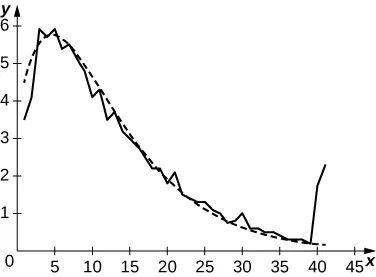239.

In dry conditions, with initial velocity $v0=30v0=30$ m/s, $D=64.3D=64.3$ and, if $v0=25,D=44.64.v0=25,D=44.64.$ In wet conditions, if $v0=30,v0=30,$ and $D=180D=180$ and if $v0=25,D=125.v0=25,D=125.$

241.

225 cal

243.

$E ( 150 ) = 28 , E ( 300 ) = 22 , E ( 450 ) = 16 E ( 150 ) = 28 , E ( 300 ) = 22 , E ( 450 ) = 16$

245.

a.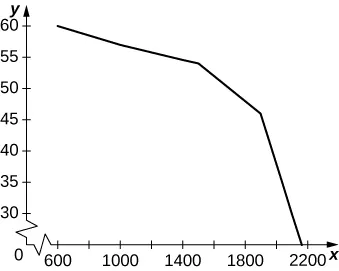b. Between 600 and 1000 the average decrease in vehicles per hour per lane is −0.0075. Between 1000 and 1500 it is −0.006 per vehicles per hour per lane, and between 1500 and 2100 it is −0.04 vehicles per hour per lane. c.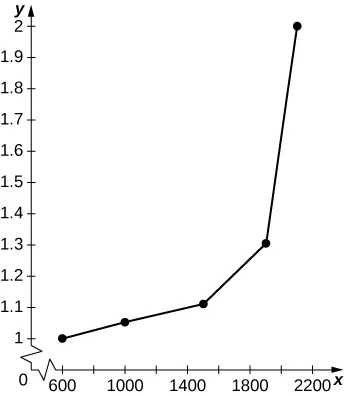The graph is nonlinear, with minutes per mile increasing dramatically as vehicles per hour per lane reach 2000.

247.

$1 37 ∫ 0 37 p ( t ) d t = 0.07 ( 37 ) 3 4 + 2.42 ( 37 ) 2 3 − 25.63 ( 37 ) 2 + 521.23 ≈ 2037 1 37 ∫ 0 37 p ( t ) d t = 0.07 ( 37 ) 3 4 + 2.42 ( 37 ) 2 3 − 25.63 ( 37 ) 2 + 521.23 ≈ 2037$

249.

Average acceleration is $A=15∫05a(t)dt=−0.7(52)3+1.44(5)2+10.44≈8.2A=15∫05a(t)dt=−0.7(52)3+1.44(5)2+10.44≈8.2$ mph/s

251.

253.

$1 40 ∫ 0 40 ( −0.068 t + 5.14 ) d t = − 0.068 ( 40 ) 2 + 5.14 = 3.78 m/sec 1 40 ∫ 0 40 ( −0.068 t + 5.14 ) d t = − 0.068 ( 40 ) 2 + 5.14 = 3.78 m/sec$

### Section 1.5 Exercises

255.

$u = h ( x ) u = h ( x )$

257.

$f ( u ) = ( u + 1 ) 2 u f ( u ) = ( u + 1 ) 2 u$

259.

$d u = 8 x d x ; f ( u ) = 1 8 u d u = 8 x d x ; f ( u ) = 1 8 u$

261.

$1 5 ( x + 1 ) 5 + C 1 5 ( x + 1 ) 5 + C$

263.

$− 1 12 ( 2 x − 3 ) 6 + C − 1 12 ( 2 x − 3 ) 6 + C$

265.

$x 2 + 1 + C x 2 + 1 + C$

267.

$1 8 ( x 2 − 2 x ) 4 + C 1 8 ( x 2 − 2 x ) 4 + C$

269.

$sin θ − sin 3 θ 3 + C sin θ − sin 3 θ 3 + C$

271.

$( 1 − x ) 101 101 − ( 1 − x ) 100 100 + C ( 1 − x ) 101 101 − ( 1 − x ) 100 100 + C$

273.

$∫(11x-7)-2dx= −122(11x−7)2+C ∫ ( 11 x - 7 ) -2 dx = − 1 22 ( 11 x − 7 ) 2 + C$

275.

$− cos 4 θ 4 + C − cos 4 θ 4 + C$

277.

$− cos 3 ( π t ) 3 π + C − cos 3 ( π t ) 3 π + C$

279.

$− 1 4 cos 2 ( t 2 ) + C − 1 4 cos 2 ( t 2 ) + C$

281.

$− 1 3 ( x 3 − 3 ) + C − 1 3 ( x 3 − 3 ) + C$

283.

$− 2 ( y 3 − 2 ) 3 1 − y 3 − 2 ( y 3 − 2 ) 3 1 − y 3$

285.

$1 33 ( 1 − cos 3 θ ) 11 + C 1 33 ( 1 − cos 3 θ ) 11 + C$

287.

$1 12 ( sin 3 θ − 3 sin 2 θ ) 4 + C 1 12 ( sin 3 θ − 3 sin 2 θ ) 4 + C$

289.

$L50=−8.5779.L50=−8.5779.$ The exact area is $−818−818$

291.

$L50=−0.006399L50=−0.006399$ … The exact area is 0.

293.

$u = 1 + x 2 , d u = 2 x d x , 1 2 ∫ 1 2 u −1 / 2 d u = 2 − 1 u = 1 + x 2 , d u = 2 x d x , 1 2 ∫ 1 2 u −1 / 2 d u = 2 − 1$

295.

$u = 1 + t 3 , d u = 3 t 2 d t , 1 3 ∫ 1 2 u −1 / 2 d u = 2 3 ( 2 − 1 ) u = 1 + t 3 , d u = 3 t 2 d t , 1 3 ∫ 1 2 u −1 / 2 d u = 2 3 ( 2 − 1 )$

297.

$u = cos θ , d u = − sin θ d θ , ∫ 1 / 2 1 u −4 d u = 1 3 ( 2 2 − 1 ) u = cos θ , d u = − sin θ d θ , ∫ 1 / 2 1 u −4 d u = 1 3 ( 2 2 − 1 )$

299.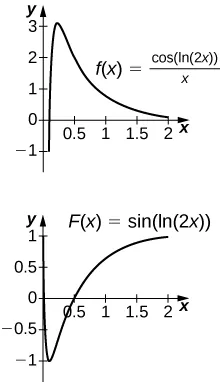The antiderivative is $y=sin(ln(2x)).y=sin(ln(2x)).$ Since the antiderivative is not continuous at $x=0,x=0,$ one cannot find a value of C that would make $y=sin(ln(2x))−Cy=sin(ln(2x))−C$ work as a definite integral.

301.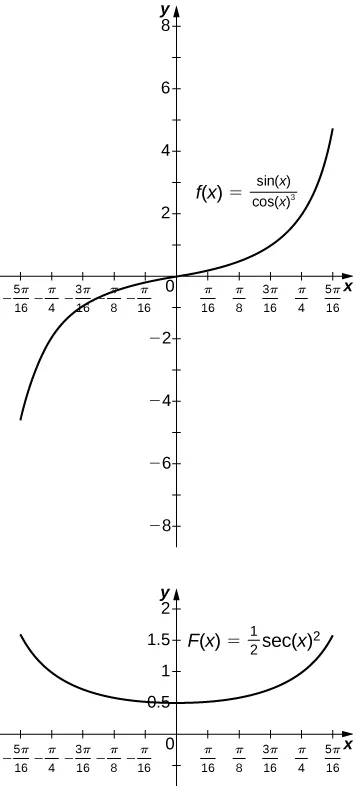The antiderivative is $y=12sec2x.y=12sec2x.$ You should take $C=−2C=−2$ so that $F(−π3)=0.F(−π3)=0.$

303.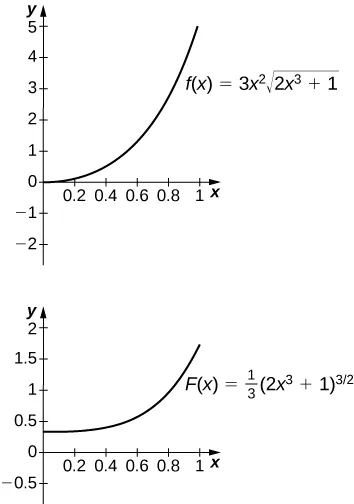The antiderivative is $y=13(2x3+1)3/2.y=13(2x3+1)3/2.$ One should take $C=−13.C=−13.$

305.

No, because the integrand is discontinuous at $x=1.x=1.$

307.

$u=sin(t2);u=sin(t2);$ the integral becomes $12∫00udu.12∫00udu.$

309.

$u=(1+(t−12)2);u=(1+(t−12)2);$ the integral becomes $−∫5/45/41udu.−∫5/45/41udu.$

311.

$u=1−t;u=1−t;$ the integral becomes
$∫1−1ucos(π(1−u))du=∫1−1u[cosπcosπu−sinπsinπu]du=−∫1−1ucosπudu=∫−11ucosπudu=0∫1−1ucos(π(1−u))du=∫1−1u[cosπcosπu−sinπsinπu]du=−∫1−1ucosπudu=∫−11ucosπudu=0$
since the integrand is odd.

313.

Setting $u=cxu=cx$ and $du=cdxdu=cdx$ gets you $1bc−ac∫a/cb/cf(cx)dx=cb−a∫u=au=bf(u)duc=1b−a∫abf(u)du.1bc−ac∫a/cb/cf(cx)dx=cb−a∫u=au=bf(u)duc=1b−a∫abf(u)du.$

315.

$∫0xg(t)dt=12∫u=1−x21duua=12(1−a)u1−a|u=1−x21=12(1−a)(1−(1−x2)1−a).∫0xg(t)dt=12∫u=1−x21duua=12(1−a)u1−a|u=1−x21=12(1−a)(1−(1−x2)1−a).$ As $x→1x→1$ the limit is $12(1−a)12(1−a)$ if $a<1,a<1,$ and the limit diverges to +∞ if $a≥1.a≥1.$

317.

$∫ t = π 0 b 1 − cos 2 t × ( − a sin t ) d t = ∫ t = 0 π a b sin 2 t d t ∫ t = π 0 b 1 − cos 2 t × ( − a sin t ) d t = ∫ t = 0 π a b sin 2 t d t$

319.

$f ( t ) = 2 cos ( 3 t ) − cos ( 2 t ) ; ∫ 0 π ( 2 cos ( 3 t ) − cos ( 2 t ) ) d t = 0 f ( t ) = 2 cos ( 3 t ) − cos ( 2 t ) ; ∫ 0 π ( 2 cos ( 3 t ) − cos ( 2 t ) ) d t = 0$

### Section 1.6 Exercises

321.

$−1 3 e −3 x + C −1 3 e −3 x + C$

323.

$− 3 − x ln 3 + C − 3 − x ln 3 + C$

325.

$2 ln x + C or ln x 2 +C 2 ln x + C or ln x 2 +C$

327.

$2 x + C 2 x + C$

329.

$− 1 ln x + C − 1 ln x + C$

331.

$ln ( ln ( ln x ) ) + C ln ( ln ( ln x ) ) + C$

333.

$ln ( x cos x ) + C ln ( x cos x ) + C$

335.

$− 1 2 ( ln ( cos ( x ) ) ) 2 + C − 1 2 ( ln ( cos ( x ) ) ) 2 + C$

337.

$− e − x 3 3 + C − e − x 3 3 + C$

339.

$e tan x + C e tan x + C$

341.

$t + C t + C$

343.

$2 9 x 3 ( ln ( x 3 ) − 1 ) + C 2 9 x 3 ( ln ( x 3 ) − 1 ) + C$

345.

$2 x ( ln x − 2 ) + C 2 x ( ln x − 2 ) + C$

347.

$∫ 0 ln x e t d t = e t | 0 ln x = e ln x − e 0 = x − 1 ∫ 0 ln x e t d t = e t | 0 ln x = e ln x − e 0 = x − 1$

349.

$- 1 3 ln sin ( 3 x ) + cos ( 3 x ) +C - 1 3 ln sin ( 3 x ) + cos ( 3 x ) +C$

351.

$− 1 2 ln | csc ( x 2 ) + cot ( x 2 ) | + C − 1 2 ln | csc ( x 2 ) + cot ( x 2 ) | + C$

353.

$− 1 2 ( ln ( csc x ) ) 2 + C − 1 2 ( ln ( csc x ) ) 2 + C$

355.

$1 3 ln ( 26 7 ) 1 3 ln ( 26 7 )$

357.

$ln ( 3 − 1 ) ln ( 3 − 1 )$

359.

$1 2 ln 3 2 1 2 ln 3 2$

361.

$y − 2 ln | y + 1 | + C y − 2 ln | y + 1 | + C$

363.

$ln | sin x − cos x | + C ln | sin x − cos x | + C$

365.

$− 1 3 ( 1 − ( ln x ) 2 ) 3 / 2 + C − 1 3 ( 1 − ( ln x ) 2 ) 3 / 2 + C$

367.

Exact solution: $e−1e,R50=0.6258.e−1e,R50=0.6258.$ Since f is decreasing, the right endpoint estimate underestimates the area.

369.

Exact solution: $2ln(3)−ln(6)2,R50=0.2033.2ln(3)−ln(6)2,R50=0.2033.$ Since f is increasing, the right endpoint estimate overestimates the area.

371.

Exact solution: $−1ln(4),R50=−0.7164.−1ln(4),R50=−0.7164.$ Since f is increasing, the right endpoint estimate overestimates the area (the actual area is a larger negative number).

373.

$11 2 ln 2 11 2 ln 2$

375.

$1 ln ( 65 , 536 ) 1 ln ( 65 , 536 )$

377.

$∫NN+1xe−x2dx=12(e−N2−e−(N+1)2).∫NN+1xe−x2dx=12(e−N2−e−(N+1)2).$ The quantity is less than 0.01 when $N=2.N=2.$

379.

$∫ a b d x x = ln ( b ) − ln ( a ) = ln ( 1 a ) − ln ( 1 b ) = ∫ 1 / b 1 / a d x x ∫ a b d x x = ln ( b ) − ln ( a ) = ln ( 1 a ) − ln ( 1 b ) = ∫ 1 / b 1 / a d x x$

381.

23

383.

We may assume that $x>1,so1x<1.x>1,so1x<1.$ Then, $∫11/xdtt.∫11/xdtt.$ Now make the substitution $u=1t,u=1t,$ so $du=−dtt2du=−dtt2$ and $duu=−dtt,duu=−dtt,$ and change endpoints: $∫11/xdtt=−∫1xduu=−lnx.∫11/xdtt=−∫1xduu=−lnx.$

385.

387.

$x=E(ln(x)).x=E(ln(x)).$ Then, $1=E'(lnx)xorx=E'(lnx).1=E'(lnx)xorx=E'(lnx).$ Since any number t can be written $t=lnxt=lnx$ for some x, and for such t we have $x=E(t),x=E(t),$ it follows that for any $t,E'(t)=E(t).t,E'(t)=E(t).$

389.

$R10=0.6811,R100=0.6827R10=0.6811,R100=0.6827$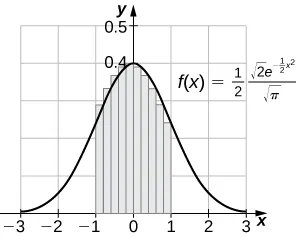### Section 1.7 Exercises

391.

$sin −1 x | 0 3 / 2 = π 3 sin −1 x | 0 3 / 2 = π 3$

393.

$tan −1 x | 3 1 = − π 12 tan −1 x | 3 1 = − π 12$

395.

$sec −1 | 2 3 2 = π 4 - π 6 = π 12 sec −1 | 2 3 2 = π 4 - π 6 = π 12$

397.

$sin −1 ( x 3 ) + C sin −1 ( x 3 ) + C$

399.

$1 3 tan −1 ( x 3 ) + C 1 3 tan −1 ( x 3 ) + C$

401.

$1 3 sec −1 ( x 3 ) + C 1 3 sec −1 ( x 3 ) + C$

403.

$cos(π2−θ)=sinθ.cos(π2−θ)=sinθ.$ So, $sin−1t=π2−cos−1t.sin−1t=π2−cos−1t.$ They differ by a constant.

405.

$1−t21−t2$ is not defined as a real number when $t>1.t>1.$

407.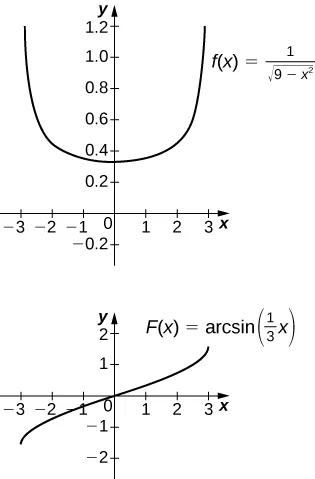The antiderivative is $sin−1(x3)+C.sin−1(x3)+C.$ Taking $C=π2C=π2$ recovers the definite integral.

409.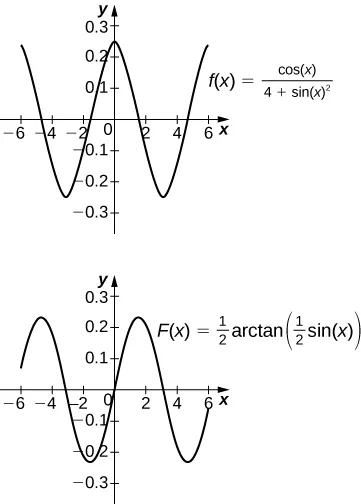The antiderivative is $12tan−1(sinx2)+C.12tan−1(sinx2)+C.$ Taking $C=12tan−1(sin(6)2)C=12tan−1(sin(6)2)$ recovers the definite integral.

411.

$1 2 ( sin −1 t ) 2 + C 1 2 ( sin −1 t ) 2 + C$

413.

$1 4 ( tan −1 ( 2 t ) ) 2 1 4 ( tan −1 ( 2 t ) ) 2$

415.

$1 4 ( sec −1 ( t 2 ) 2 ) + C 1 4 ( sec −1 ( t 2 ) 2 ) + C$

417.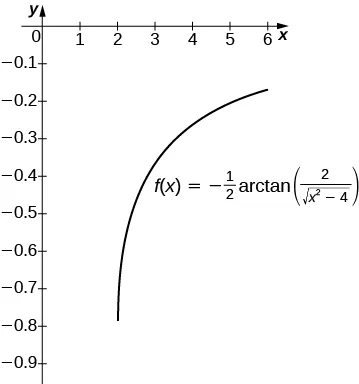The antiderivative is $12sec−1(x2)+C.12sec−1(x2)+C.$ Taking $C=0C=0$ recovers the definite integral over $[2,6].[2,6].$

419.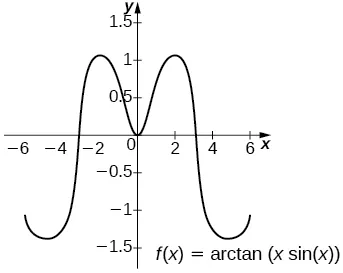The general antiderivative is $tan−1(xsinx)+C.tan−1(xsinx)+C.$ Taking $C=−tan−1(6sin(6))C=−tan−1(6sin(6))$ recovers the definite integral.

421.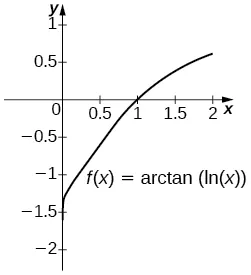The general antiderivative is $tan−1(lnx)+C.tan−1(lnx)+C.$ Taking $C=π2=tan−1∞C=π2=tan−1∞$ recovers the definite integral.

423.

$sin −1 ( e t ) + C sin −1 ( e t ) + C$

425.

$sin −1 ( ln t ) + C sin −1 ( ln t ) + C$

427.

$− 1 4 ( cos −1 ( 2 t ) ) 2 + C − 1 4 ( cos −1 ( 2 t ) ) 2 + C$

429.

$1 2 ln ( 4 3 ) 1 2 ln ( 4 3 )$

431.

$1 − 2 5 1 − 2 5$

433.

$2tan−1(A)→π2tan−1(A)→π$ as $A→∞A→∞$

435.

Using the hint, one has $∫csc2xcsc2x+cot2xdx=∫csc2x1+2cot2xdx.∫csc2xcsc2x+cot2xdx=∫csc2x1+2cot2xdx.$ Set $u=2cotx.u=2cotx.$ Then, $du=−2csc2xdu=−2csc2x$ and the integral is $−12∫du1+u2=−12tan−1u+C=12tan−1(2cotx)+C.−12∫du1+u2=−12tan−1u+C=12tan−1(2cotx)+C.$ If one uses the identity $tan−1s+tan−1(1s)=π2,tan−1s+tan−1(1s)=π2,$ then this can also be written $12tan−1(tanx2)+C.12tan−1(tanx2)+C.$

437.

$x≈±1.7321.x≈±1.7321.$ The left endpoint estimate with $N=100N=100$ is 4.781 and these decimals persist for $N=500.N=500.$

### Review Exercises

439.

False

441.

True

443.

$L4=5.25,R4=3.25,L4=5.25,R4=3.25,$ exact answer: 4

445.

$L4=5.364,R4=5.364,L4=5.364,R4=5.364,$ exact answer: 5.870

447.

$− 4 3 − 4 3$

449.

1

451.

$− 1 2 ( x + 4 ) 2 + C − 1 2 ( x + 4 ) 2 + C$

453.

$4 3 sin −1 ( x 3 ) + C 4 3 sin −1 ( x 3 ) + C$

455.

$sin t 1 + t 2 sin t 1 + t 2$

457.

$4 ln x x + 1 4 ln x x + 1$

459.

$6,328,113 461.$73.36

463.

$19117 12 ft/sec , or 1593 ft/sec 19117 12 ft/sec , or 1593 ft/sec$

Order a print copy

As an Amazon Associate we earn from qualifying purchases.